# Tutorial 3: Regions and Property Specifications¶

Context

In this tutorial, we set up a simple field case for single-phase flow simulation (see Singlephase Flow Solver). We demonstrate how to run a basic flow simulation in the reservoir layer. We do not consider any coupling with wells. Injection and production will be specified by imposing a high pressure in the cells close to the injection area and a low pressure in the cells close to the production area.

Objectives

At the end of this tutorial you will know:

• how to import external mesh information and properties,

• how to run a specific solver (here, flow) in a specific region only,

• the basic method of using boxes to set up boundary conditions,

• how to use TableFunction to import fields varying in time and/or space,

• how to control output frequency and export results for visualization.

Input file

The XML input file for this test case is located at:

```inputFiles/singlePhaseFlow/FieldCaseTutorial3_base.xml
```
```inputFiles/singlePhaseFlow/FieldCaseTutorial3_smoke.xml
```

We consider the following mesh as a numerical support to the simulations in this tutorial: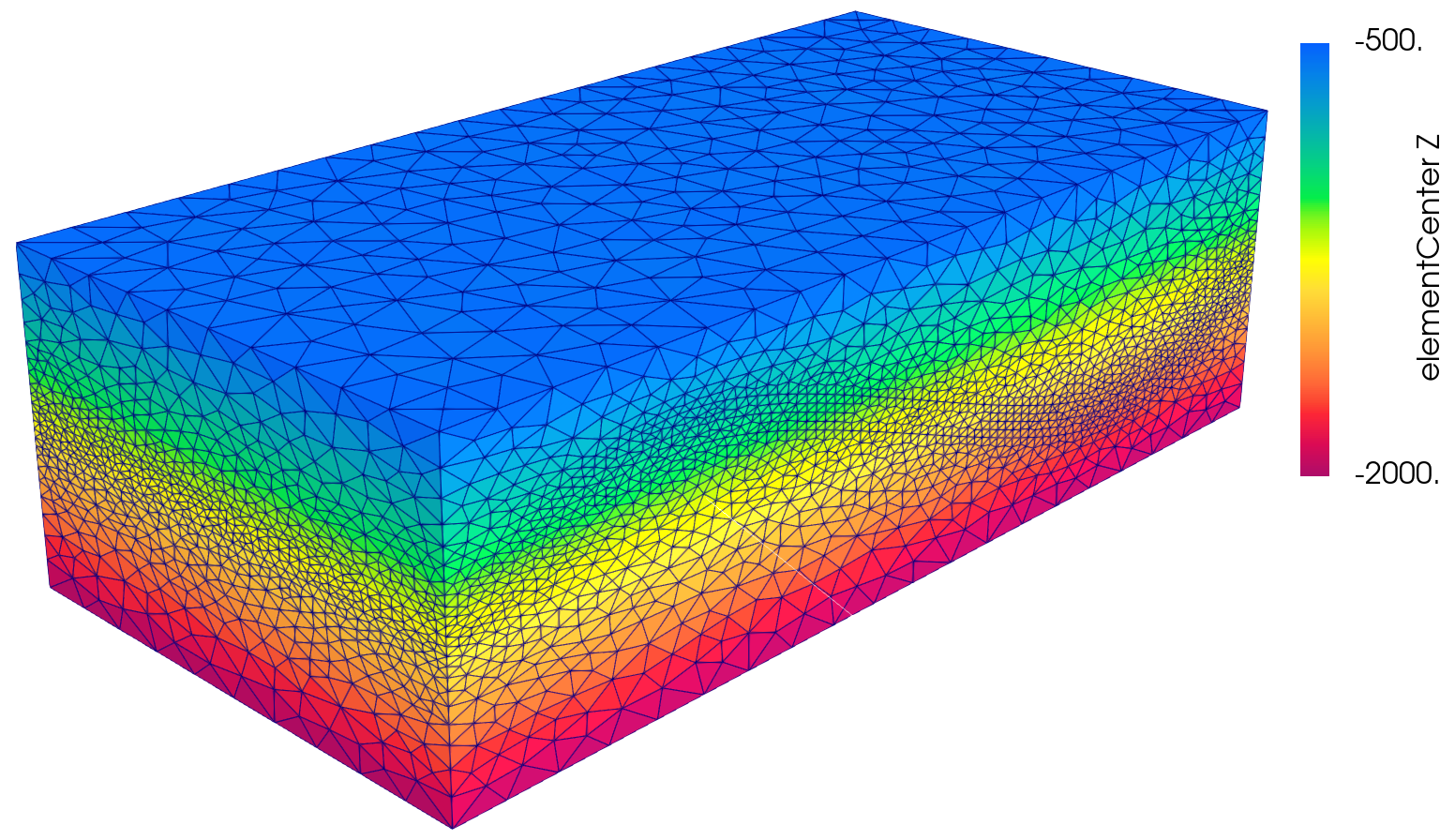This mesh contains three continuous regions:

• a Top region (overburden, elementary tag = Overburden)

• a Middle region (reservoir layer, elementary tag = Reservoir)

• a Bottom region (underburden, elementary tag = Underburden)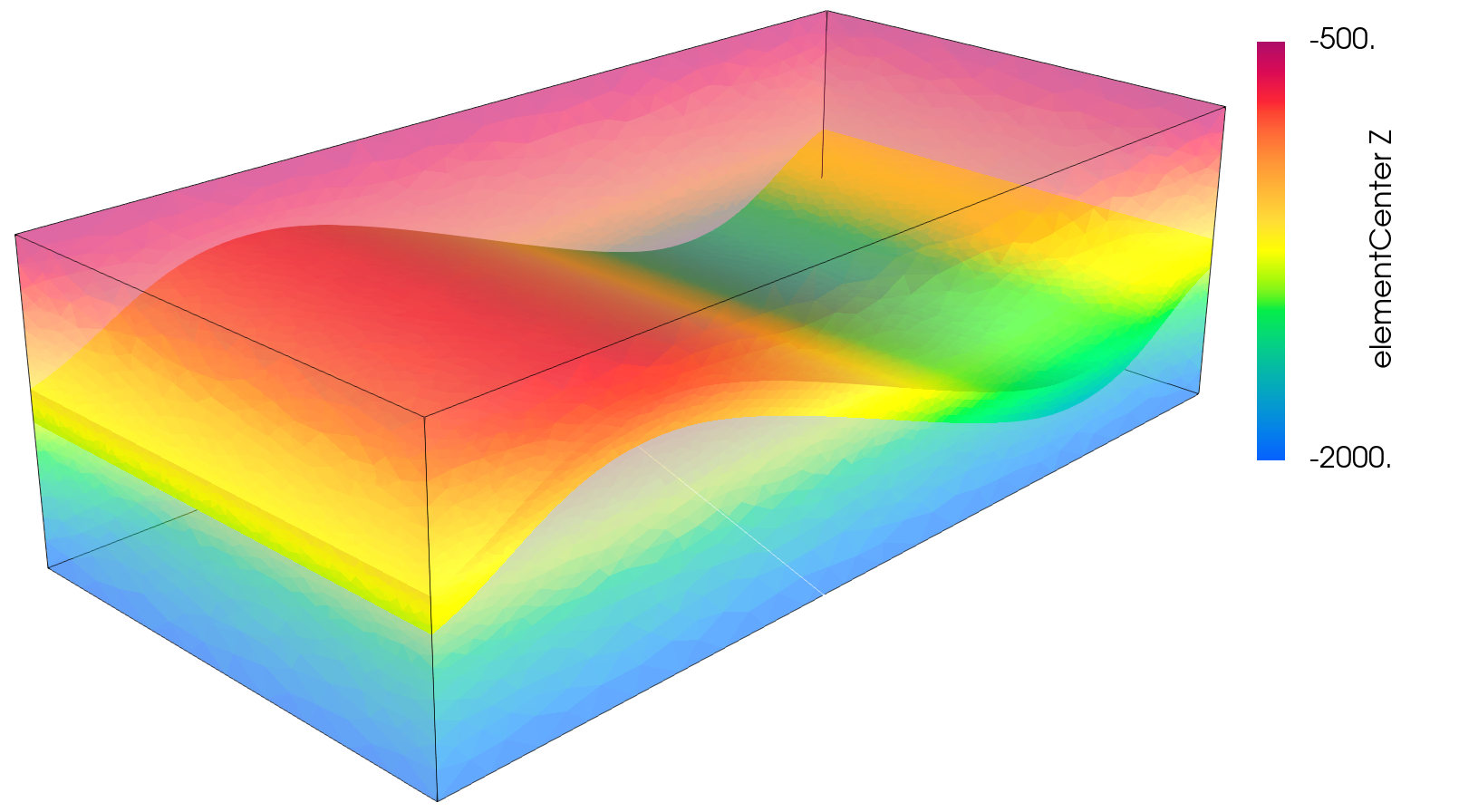The mesh is defined using the VTK file format (see Meshes for more information on the supported mesh file format). Each tetrahedron is associated to a unique tag.

The XML file considered here follows the typical structure of the GEOS input files:

## Single-phase solver¶

Let us inspect the Solver XML tags.

```  <Solvers>
<SinglePhaseFVM
name="SinglePhaseFlow"
discretization="singlePhaseTPFA"
targetRegions="{ Reservoir }">
<NonlinearSolverParameters
newtonTol="1.0e-6"
newtonMaxIter="8"/>
<LinearSolverParameters
solverType="gmres"
preconditionerType="amg"
amgSmootherType="l1jacobi"
krylovTol="1.0e-10"/>
</SinglePhaseFVM>
</Solvers>
```

This node gathers all the information previously defined. We use a classical `SinglePhaseFVM` Finite Volume Method, with the two-point flux approximation as will be defined in the NumericalMethods tag. The `targetRegions` refers only to the Reservoir region because we only solve for flow in this region.

The `NonlinearSolverParameters` and `LinearSolverParameters` are used to set usual numerical solver parameters such as the linear and nonlinear tolerances, the preconditioner and solver types or the maximum number of nonlinear iterations.

## Mesh¶

Here, we use the `VTKMesh` to load the mesh (see Importing the Mesh). The syntax to import external meshes is simple : in the XML file, the mesh `file` is included with its relative or absolute path to the location of the GEOS XML file and a user-specified `name` label for the mesh object.

```  <Mesh>
<VTKMesh name="SyntheticMesh"
file="synthetic.vtu" />
</Mesh>
```

## Geometry¶

Here, we are using definition of `source` and `sink` boxes in addition to the `all` box in order to flag sets of nodes or cells which will act as injection or production.

```  <Geometry>
<Box
name="all"
xMin="{ -1e9, -1e9, -1e9 }"
xMax="{ 1e9, 1e9, 1e9 }"/>

<Box
name="source"
xMin="{ 15500, 7000, -5000 }"
xMax="{ 16000, 7500, 0 }"/>

<Box
name="sink"
xMin="{ 6500, 1500, -5000 }"
xMax="{ 7000, 2000, 0 }"/>
</Geometry>
```

In order to define a box, the user defines `xMax` and `xMin`, two diagonally opposite nodes of the box.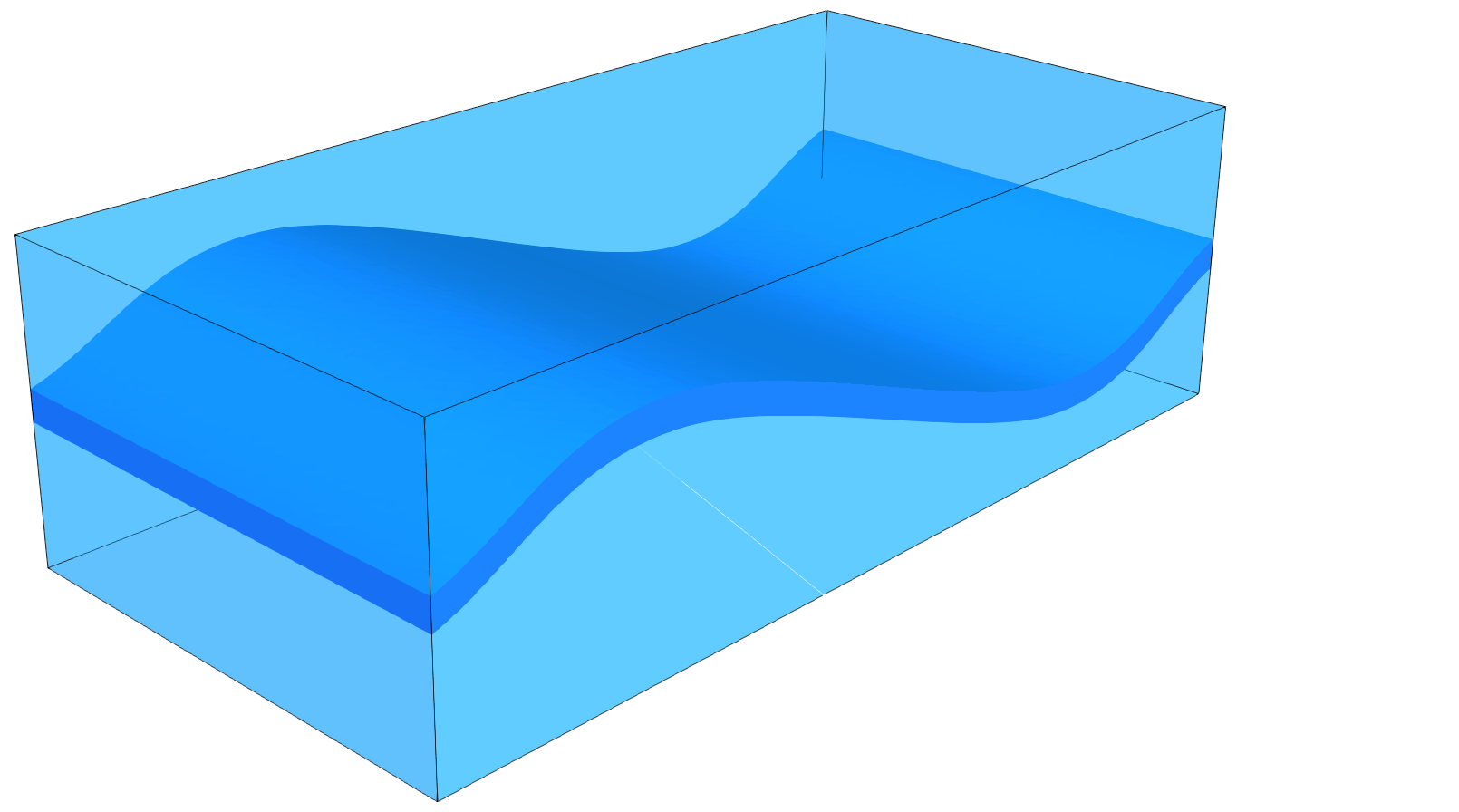## Events¶

The events are used here to guide the simulation through time, and specify when outputs must be triggered.

```  <Events maxTime="100.0e6">
<PeriodicEvent name="solverApplications"
forceDt="10.0e6"
target="/Solvers/SinglePhaseFlow" />

<PeriodicEvent name="outputs"
timeFrequency="10.0e6"
target="/Outputs/reservoir_with_properties" />
</Events>
```

The Events tag is associated with the `maxTime` keyword defining the maximum time. If this time is ever reached or exceeded, the simulation ends.

Two `PeriodicEvent` are defined. - The first one, `solverApplications`, is associated with the solver. The `forceDt` keyword means that there will always be time-steps of 10e6 seconds. - The second, `outputs`, is associated with the output. The `timeFrequency` keyword means that it will be executed every 10e6 seconds.

## Numerical methods¶

Defining the numerical method used in the solver, we will provide information on how to discretize our equations. Here a classical two-point flux approximation (TPFA) scheme is used to discretize water fluxes over faces.

```  <NumericalMethods>
<FiniteVolume>
<TwoPointFluxApproximation
name="singlePhaseTPFA"
/>
</FiniteVolume>
</NumericalMethods>
```

## Regions¶

Assuming that the overburden and the underburden are impermeable, and flow only takes place in the reservoir, we need to define regions.

There are two methods to achieve this regional solve.

• The first solution is to define a unique `CellElementRegion` corresponding to the reservoir.

```<ElementRegions>
<CellElementRegion
name="Reservoir"
cellBlocks="{2_tetrahedra}"
materialList="{ water, rock, rockPerm, rockPorosity, nullSolid }"/>
</ElementRegions>
```
• The second solution is to define all the `CellElementRegions` as they are in the VTK file, but defining the solvers only on the reservoir layer. In this case, the ElementRegions tag is :

```  <ElementRegions>
<CellElementRegion
name="Reservoir"
cellBlocks="{ 2_tetrahedra }"
materialList="{ water, rock }"/>

<CellElementRegion
name="Burden"
cellBlocks="{ 1_tetrahedra, 3_tetrahedra }"
materialList="{ water, rock }"/>
</ElementRegions>
```

We opt for the latest as it allows to visualize over- and underburdens and to change regions handling in their tag without needing to amend the ElementRegion tag.

Note

The material list here was set for a single-phase flow problem. This list is subject to change if the problem is not a single-phase flow problem.

## Constitutive models¶

We simulate a single-phase flow in the reservoir layer, hence with multiple types of materials, a fluid (water) and solid (rock permeability and porosity).

```  <Constitutive>
<CompressibleSinglePhaseFluid
name="water"
defaultDensity="1000"
defaultViscosity="0.001"
referencePressure="0.0"
compressibility="1e-9"
viscosibility="0.0"/>

<CompressibleSolidConstantPermeability
name="rock"
solidModelName="nullSolid"
porosityModelName="rockPorosity"
permeabilityModelName="rockPerm"/>

<NullModel
name="nullSolid"/>

<PressurePorosity
name="rockPorosity"
defaultReferencePorosity="0.05"
referencePressure="10e7"
compressibility="1.0e-9"/>

<ConstantPermeability
name="rockPerm"
permeabilityComponents="{ 1.0e-13, 1.0e-13, 1.0e-16 }"/>
</Constitutive>
```

The constitutive parameters such as the density, the viscosity, and the compressibility are specified in the International System of Units.

Note

To consider an incompressible fluid, the user has to set the compressibility to 0.

Note

GEOS handles permeability as a diagonal matrix, so the three values of the permeability tensor are set individually using the `component` field.

## Defining properties¶

The next step is to specify fields, including:

• The initial value (here, the pressure has to be initialized)

• The static properties (here, we have to define the permeability tensor and the porosity)

• The boundary conditions (here, the injection and production pressure have to be set)

```  <FieldSpecifications>

<FieldSpecification
name="permx"
initialCondition="1"
component="0"
setNames="{ all }"
objectPath="ElementRegions/Reservoir"
fieldName="rockPerm_permeability"
scale="1e-15"
functionName="permxFunc"/>

<FieldSpecification
name="permy"
initialCondition="1"
component="1"
setNames="{ all }"
objectPath="ElementRegions/Reservoir"
fieldName="rockPerm_permeability"
scale="1e-15"
functionName="permyFunc"/>

<FieldSpecification
name="permz"
initialCondition="1"
component="2"
setNames="{ all }"
objectPath="ElementRegions/Reservoir"
fieldName="rockPerm_permeability"
scale="3e-15"
functionName="permzFunc"/>

<FieldSpecification
name="initialPressure"
initialCondition="1"
setNames="{ all }"
objectPath="ElementRegions/Reservoir/2_tetrahedra"
fieldName="pressure"
scale="1e7"
/>

<FieldSpecification
name="sourceTerm"
objectPath="ElementRegions/Reservoir/2_tetrahedra"
fieldName="pressure"
scale="15e7"
setNames="{ source }"
/>

<FieldSpecification
name="sinkTerm"
objectPath="ElementRegions/Reservoir/2_tetrahedra"
fieldName="pressure"
scale="5e7"
setNames="{ sink }"/>
</FieldSpecifications>
```

You may note :

• All static parameters and initial value fields must have `initialCondition` field set to `1`.

• The `objectPath` refers to the `ElementRegion` in which the field has its value,

• The `setName` field points to the box previously defined to apply the fields,

• `name` and `fieldName` have a different meaning: `name` is used to give a name to the XML block. This `name` must be unique. `fieldName` is the name of the field registered in GEOS. This value has to be set according to the expected input fields of each solver.

## Output¶

The Outputs XML tag is used to trigger the writing of visualization files. Here, we write files in a format natively readable by Paraview under the tag VTK:

```  <Outputs>
<VTK
name="reservoir_with_properties"/>
</Outputs>
```

Note

The `name` keyword defines the name of the output directory.

## Using functions to specify properties¶

Eventually, one can define varying properties using `TableFunction` (Functions) under the Functions tag:

```  <Functions>
<TableFunction
name="timeInj"
inputVarNames="{ time }"
coordinates="{ 1e6, 10e6, 50e6 }"
values="{ 1, 0.01, 0.00001 }"/>

<TableFunction
name="initialPressureFunc"
inputVarNames="{ elementCenter }"
coordinateFiles="{ tables_FieldCaseTuto/xlin.geos, tables_FieldCaseTuto/ylin.geos, tables_FieldCaseTuto/zlin.geos }"
voxelFile="tables_FieldCaseTuto/pressure.geos"/>

<TableFunction
name="permxFunc"
inputVarNames="{ elementCenter }"
coordinateFiles="{ tables_FieldCaseTuto/xlin.geos, tables_FieldCaseTuto/ylin.geos, tables_FieldCaseTuto/zlin.geos }"
voxelFile="tables_FieldCaseTuto/permx.geos"
interpolation="nearest"/>

<TableFunction
name="permyFunc"
inputVarNames="{ elementCenter }"
coordinateFiles="{ tables_FieldCaseTuto/xlin.geos, tables_FieldCaseTuto/ylin.geos, tables_FieldCaseTuto/zlin.geos }"
voxelFile="tables_FieldCaseTuto/permy.geos"
interpolation="nearest"/>

<TableFunction
name="permzFunc"
inputVarNames="{ elementCenter }"
coordinateFiles="{ tables_FieldCaseTuto/xlin.geos, tables_FieldCaseTuto/ylin.geos, tables_FieldCaseTuto/zlin.geos }"
voxelFile="tables_FieldCaseTuto/permz.geos"
interpolation="nearest"/>
</Functions>
```

Here, the injection pressure is set to vary with time. Attentive reader might have noticed that `sourceTerm` was bound to a `TableFunction` named timeInj under FieldSpecifications tag definition. The initial pressure is set based on the values contained in the table formed by the files which are specified. In particular, the files xlin.geos, ylin.geos and zlin.geos define a regular meshing of the bounding box containing the reservoir. The pressure.geos file then defines the values of the pressure at those points.

We proceed in a similar manner as for pressure.geos to map a heterogeneous permeability field (here the 5th layer of the SPE 10 test case) onto our unstructured grid. This mapping will use a nearest point interpolation rule.Note

The varying values imposed in values or passed through voxelFile are premultiplied by the scale attribute from FieldSpecifications.

## Running GEOS¶

The simulation can be launched with:

```geosx -i FieldCaseTutorial3_smoke.xml
```

One can notice the correct load of the field function among the starting output messages

```Adding Mesh: VTKMesh, SyntheticMesh
Adding Solver of type SinglePhaseFVM, named SinglePhaseFlow
TableFunction: timeInj
TableFunction: initialPressureFunc
TableFunction: permxFunc
TableFunction: permyFunc
TableFunction: permzFunc
Adding Object CellElementRegion named Reservoir from ObjectManager::Catalog.
Adding Object CellElementRegion named Burden from ObjectManager::Catalog.
```

## Visualization of results¶

We can open the file syntheticReservoirVizFile.pvd with Paraview to visualize the simulation results. In the event block, we have asked for the output to be generated at regular intervals throughout the simulation, we can thus visualize the pressure distribution at different simulation times, showing the variation in the injection control.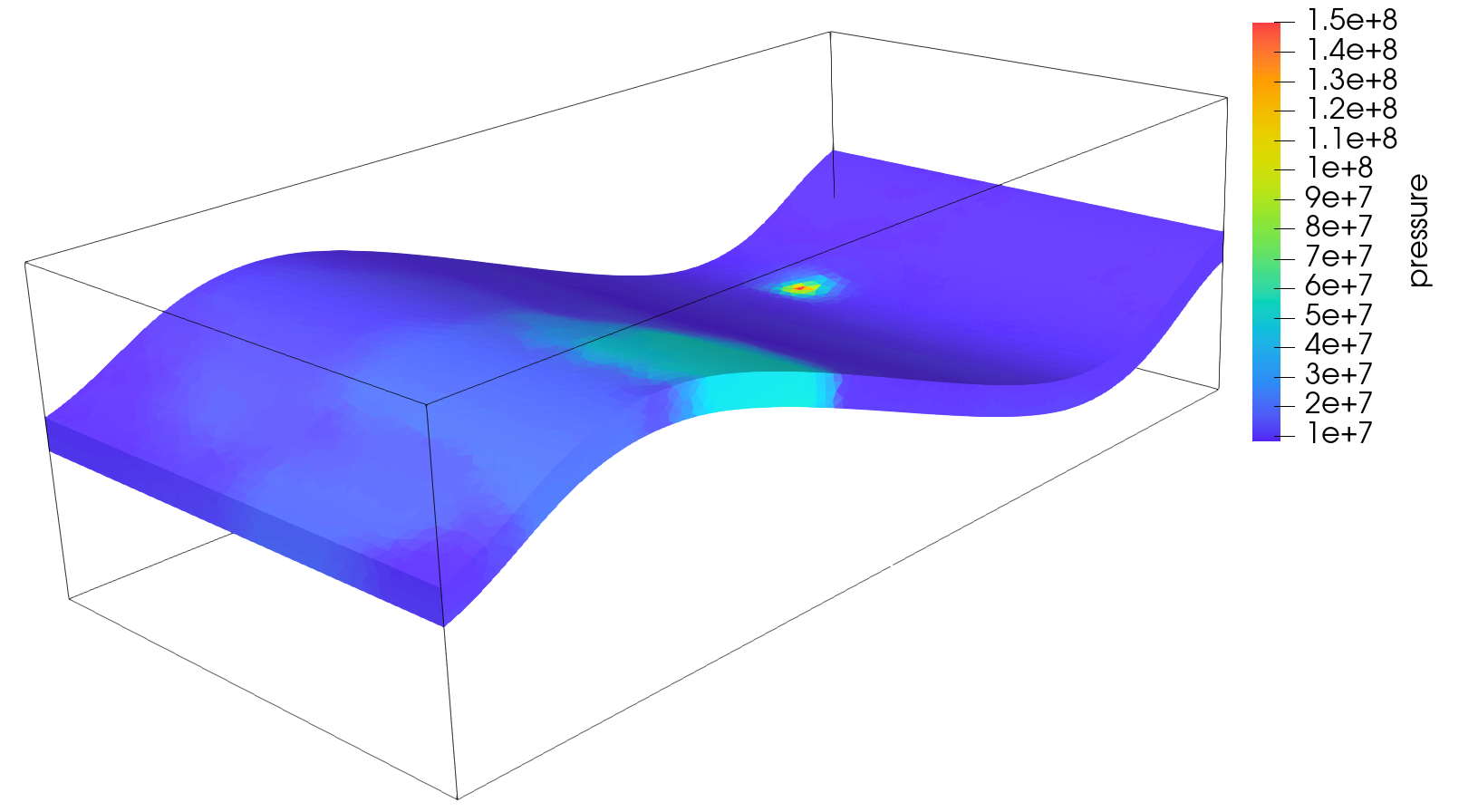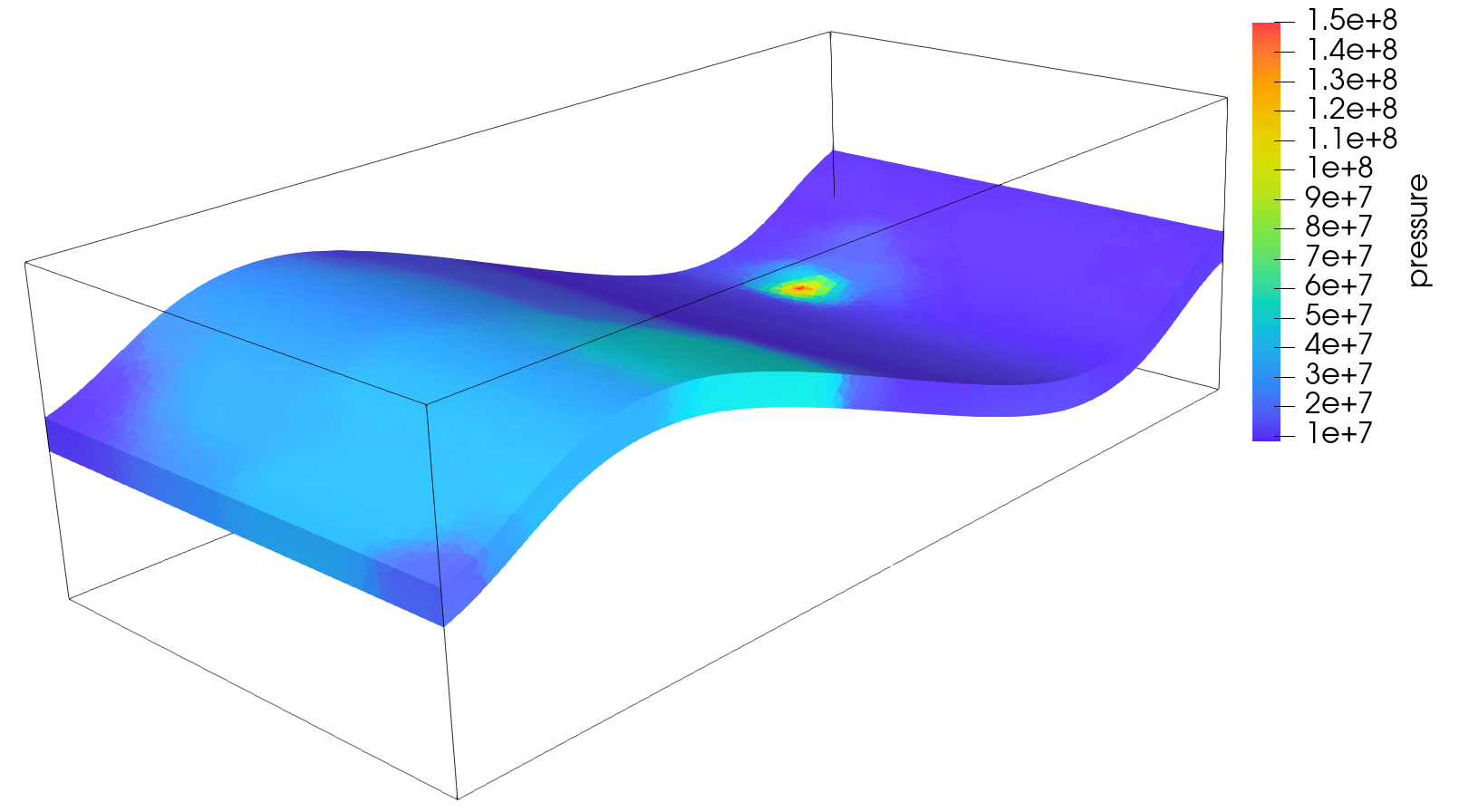## To go further¶

Feedback on this tutorial

This concludes this tutorial. For any feedback, please submit a GitHub issue on the project’s GitHub page.

For more details